2019-04-29 20:28:34 qq_37998081 阅读数 56
• ###### 图解Java数据结构和算法

1.算法是程序的灵魂，优秀的程序在对海量数据处理时，依然保持高速计算，就需要高效的数据结构和算法支撑。2.网上数据结构和算法的课程不少，但存在两个问题： 1)授课方式单一，大多是照着代码念一遍，数据结构和算法本身就比较难理解，对基础好的学员来说，还好一点，对基础不好的学生来说，基本上就是听天书了 2)说是讲数据结构和算法，但大多是挂羊头卖狗肉，算法讲的很少。 本课程针对上述问题，有针对性的进行了升级  3)授课方式采用图解+算法游戏的方式，让课程生动有趣好理解 4)系统全面的讲解了数据结构和算法, 除常用数据结构和算法外，还包括程序员常用10大算法：二分查找算法（非递归）、分治算法、动态规划算法、KMP算法、贪心算法、普里姆算法、克鲁斯卡尔算法、迪杰斯特拉算法、弗洛伊德算法、马踏棋盘算法。可以解决面试遇到的最短路径、最小生成树、最小连通图、动态规划等问题及衍生出的面试题，让你秒杀其他面试小伙伴 3.如果你不想永远都是代码工人，就需要花时间来研究下数据结构和算法。教程内容： 本教程是使用Java来讲解数据结构和算法，考虑到数据结构和算法较难，授课采用图解加算法游戏的方式。内容包括: 稀疏数组、单向队列、环形队列、单向链表、双向链表、环形链表、约瑟夫问题、栈、前缀、中缀、后缀表达式、中缀表达式转换为后缀表达式、递归与回溯、迷宫问题、八皇后问题、算法的时间复杂度、冒泡排序、选择排序、插入排序、快速排序、归并排序、希尔排序、基数排序(桶排序)、堆排序、排序速度分析、二分查找、插值查找、斐波那契查找、散列、哈希表、二叉树、二叉树与数组转换、二叉排序树(BST)、AVL树、线索二叉树、赫夫曼树、赫夫曼编码、多路查找树(B树B+树和B*树)、图、图的DFS算法和BFS、程序员常用10大算法、二分查找算法(非递归)、分治算法、动态规划算法、KMP算法、贪心算法、普里姆算法、克鲁斯卡尔算法、迪杰斯特拉算法、弗洛伊德算法马踏棋盘算法。 学习目标：通过学习，学员能掌握主流数据结构和算法的实现机制，开阔编程思路，提高优化程序的能力。

5213 人正在学习 去看看 佟刚

# 大话数据结构之串与模式匹配KMP

2019.4.29

``````typedef char String[MAXSIZE+1]; /*  0号单元存放串的长度 */
// init
Status StrAssign(String T,char *chars)
{
int i;
if(strlen(chars)>MAXSIZE)
return ERROR;
else
{
T=strlen(chars);
for(i=1;i<=T;i++)
T[i]=*(chars+i-1);
return OK;
}
}

//strcopy
Status StrCopy(String T,String S)
{
int i;
for(i=0;i<=S;i++)
T[i]=S[i];
return OK;
}

//empty?
Status StrEmpty(String S)
{
if(S==0)
return TRUE;
else
return FALSE;
}

//strcompare
//clear
//length
//strcat
// substring
//index
//insert
//sredelete
//replace
``````
• 模式匹配
``````//朴素模式匹配
int Index(String S, String T, int pos)
{
int i = pos;	/* i用于主串S中当前位置下标值，若pos不为1，则从pos位置开始匹配 */
int j = 1;				/* j用于子串T中当前位置下标值 */
while (i <= S && j <= T) /* 若i小于S的长度并且j小于T的长度时，循环继续 */
{
if (S[i] == T[j]) 	/* 两字母相等则继续 */
{
++i;
++j;
}
else 				/* 指针后退重新开始匹配 */
{
i = i-j+2;		/* i退回到上次匹配首位的下一位 */
j = 1; 			/* j退回到子串T的首位 */
}
}
if (j > T)
return i-T;
else
return 0;
}

//KMP
//getnext
void get_next(String T, int *next)
{
int i,j;
i=1;
j=0;
next=0;
while (i<T)  /* 此处T表示串T的长度 */
{
if(j==0 || T[i]== T[j]) 	/* T[i]表示后缀的单个字符，T[j]表示前缀的单个字符 */
{
++i;
++j;
next[i] = j;
}
else
j= next[j];	/* 若字符不相同，则j值回溯 */
}
}

//kmp
int Index_KMP(String S, String T, int pos)
{
int i = pos;		/* i用于主串S中当前位置下标值，若pos不为1，则从pos位置开始匹配 */
int j = 1;			/* j用于子串T中当前位置下标值 */
int next;		/* 定义一next数组 */
get_next(T, next);	/* 对串T作分析，得到next数组 */
while (i <= S && j <= T) /* 若i小于S的长度并且j小于T的长度时，循环继续 */
{
if (j==0 || S[i] == T[j]) 	/* 两字母相等则继续，与朴素算法增加了j=0判断 */
{
++i;
++j;
}
else 			/* 指针后退重新开始匹配 */
j = next[j];/* j退回合适的位置，i值不变 */
}
if (j > T)
return i-T;
else
return 0;
}

``````

kmp 大话数据结构 相关内容

2018-08-24 23:30:46 qq_36770641 阅读数 456
• ###### 图解Java数据结构和算法

1.算法是程序的灵魂，优秀的程序在对海量数据处理时，依然保持高速计算，就需要高效的数据结构和算法支撑。2.网上数据结构和算法的课程不少，但存在两个问题： 1)授课方式单一，大多是照着代码念一遍，数据结构和算法本身就比较难理解，对基础好的学员来说，还好一点，对基础不好的学生来说，基本上就是听天书了 2)说是讲数据结构和算法，但大多是挂羊头卖狗肉，算法讲的很少。 本课程针对上述问题，有针对性的进行了升级  3)授课方式采用图解+算法游戏的方式，让课程生动有趣好理解 4)系统全面的讲解了数据结构和算法, 除常用数据结构和算法外，还包括程序员常用10大算法：二分查找算法（非递归）、分治算法、动态规划算法、KMP算法、贪心算法、普里姆算法、克鲁斯卡尔算法、迪杰斯特拉算法、弗洛伊德算法、马踏棋盘算法。可以解决面试遇到的最短路径、最小生成树、最小连通图、动态规划等问题及衍生出的面试题，让你秒杀其他面试小伙伴 3.如果你不想永远都是代码工人，就需要花时间来研究下数据结构和算法。教程内容： 本教程是使用Java来讲解数据结构和算法，考虑到数据结构和算法较难，授课采用图解加算法游戏的方式。内容包括: 稀疏数组、单向队列、环形队列、单向链表、双向链表、环形链表、约瑟夫问题、栈、前缀、中缀、后缀表达式、中缀表达式转换为后缀表达式、递归与回溯、迷宫问题、八皇后问题、算法的时间复杂度、冒泡排序、选择排序、插入排序、快速排序、归并排序、希尔排序、基数排序(桶排序)、堆排序、排序速度分析、二分查找、插值查找、斐波那契查找、散列、哈希表、二叉树、二叉树与数组转换、二叉排序树(BST)、AVL树、线索二叉树、赫夫曼树、赫夫曼编码、多路查找树(B树B+树和B*树)、图、图的DFS算法和BFS、程序员常用10大算法、二分查找算法(非递归)、分治算法、动态规划算法、KMP算法、贪心算法、普里姆算法、克鲁斯卡尔算法、迪杰斯特拉算法、弗洛伊德算法马踏棋盘算法。 学习目标：通过学习，学员能掌握主流数据结构和算法的实现机制，开阔编程思路，提高优化程序的能力。

5213 人正在学习 去看看 佟刚

## 1、串的匹配是串的基础同时也非常重要的操作，最初人们用朴素的模式匹配算法，即：

``````int Index(String S, String T, int pos){    //在主串S中找到从pos开始与子串T相匹配的第一个字符的位置
int i=pos;
int j=1;
while(i<=S&&j<=T){    // 注意i<=S和j<=T要同时满足
if(S[i]==T[j]){    //匹配一致，则均后移继续匹配
i++;
j++;
}
else{        //匹配不一致，则主串下标后移一位，子串下标置1
i=i-j+2;
j=1;
}
}
if(j>T)    //j>T说明子串全部比较完且与主串中某一部分一致
return i-T;	// 注意i才是主串的下标，i-T返回开始匹配的位置
else
return 0;
}
``````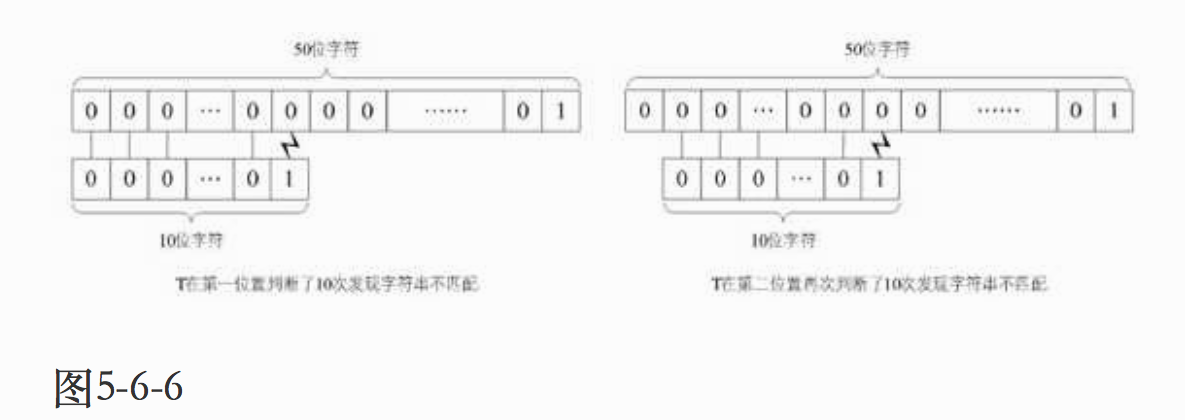## 2、KMP模式匹配算法发现了朴素算法的不足，主要特点是：

### 2）、待匹配串中存在回文相等，且它们在主串中有对应的子串，当后一位匹配失败时，待匹配串中回文串的前几位不必再与主串中明显与自己相等的字符去比较。

``````// 通过计算返回子串T的next数组
void get_next(String T, int *next){
int i,j;
i=1;
j=0;
next=0;
// 此处T表示串T的长度
while(i<T){
// T[i]表示后缀的单个字符
// T[j]表示前缀的单个字符
if(j==0 || T[i]==T[j]){
++i;
++j;
next[i]=j;
}
else
// 若字符不相同，则j值回溯
j=next[j];
}
}

// 返回子串T在主串S中第pos个字符之后的位置。
// 若不存在，则函数返回值为0
// T非空，1<=pos<=StrLength(S)
int Index_KMP(String S, String T, int pos){
// i 用于主串S当前位置下标值，若pos不为1，则从pos位置开始匹配
int i=pos;
//j 用于子串T中当前位置下标值
int j=1;
// 定义next数组
int next;

// 对串T作分析，得到next数组
get_next(T,next);

// 若i小于S的长度且j小于T的长度时，循环继续
while(i<=S && j<=T){
// 两字母相等则继续，相对于朴素算法增加了
// j=0 判断
if(j==0||S[i]==T[j]){
++i;
++j;
}
// 指针后退重新开始匹配
else{
// j退回合适的位置，i值不变
j=next[j];
}
}
if(j>T){
return i-T;
}
else
return 0;
}
``````

## 3、KMP模式匹配算法的改进：

### 2）、若当前字符与前缀字符相同，则将前缀字符的nextval值赋值给nextval在i位置的值。

``````//KMP模式匹配算法的改进

// 求模式串T的next函数修正值并存入数组nextval
void get_nextval(String T,int *nextval){
int i,j;
i=1;
j=0;
nextval=0;
while(i<T){
if(j==0||T[i]==T[j]){
i++;
j++;
// 若当前字符与前缀字符不同
if(T[i]!=T[j])
// 则当前的j为nextval在i位置的值
nextval[i]=j;
else
//如果与前缀字符相同，则将前缀字符的nextval值赋值给nextval在i位置的值
nextval[i]=nextval[j];
}
else
//若字符不相同，则j值回溯
j=nextval[j];
}
}``````

－－－－－－－－－－－－－－－－－－－－－－－－－－－－－－－－－－－－－－－－－－－－－－－－－－－－－－－－

# 概念引入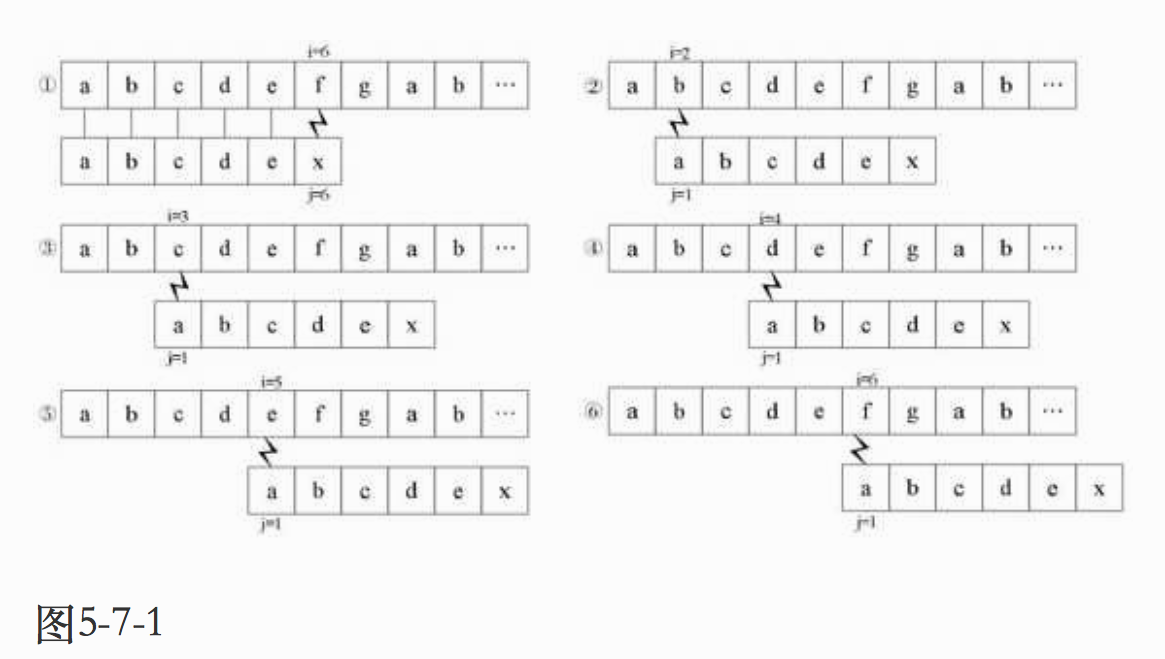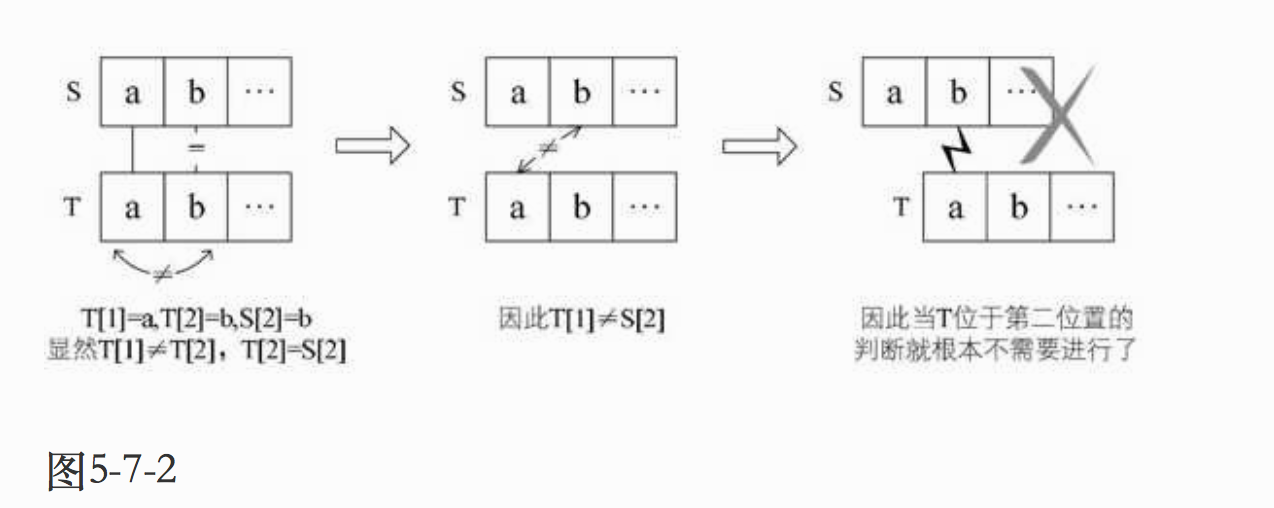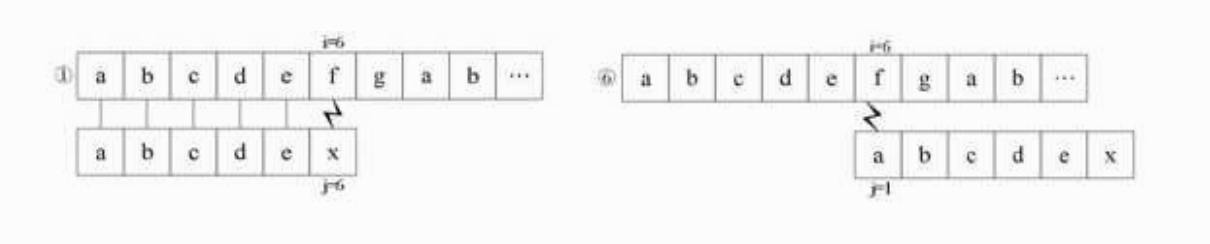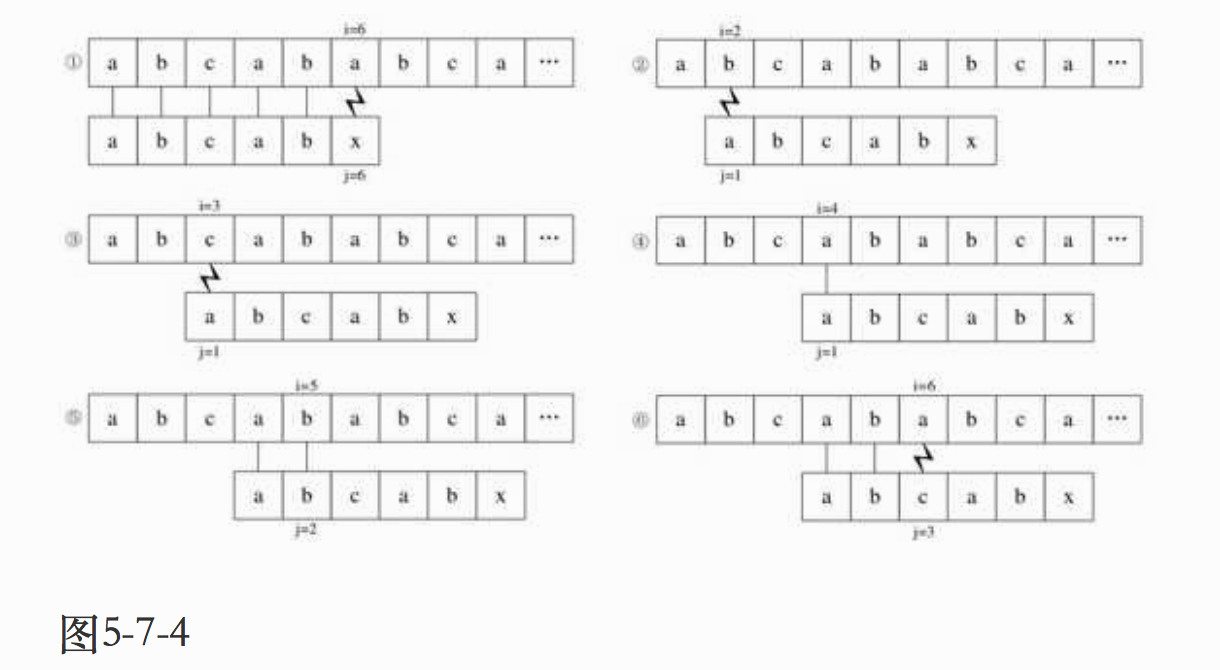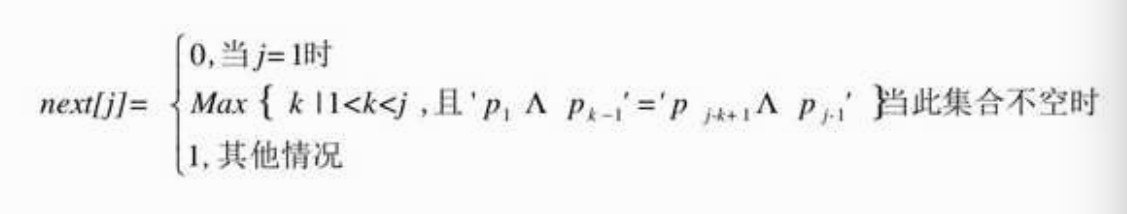# next数组推导

1．T="abcdex"

j          123456

next[j]        011111
1）当j=1时，next=0；

2）当j=2时，j由1到j-1就只有字符“a”，属于其他情况next=1；

3）当j=3时，j由1到j-1串是“ab”，显然“a”与“b”不相等，属其他情况，next=1；

4）以后同理，所以最终此T串的next[j]为011111。

2．T="abcabx"

j          123456

next[j]       011123
1）当j=1时，next=0；

2）当j=2时，同上例说明，next=1；

3）当j=3时，同上，next=1；

4）当j=4时，同上，next=1；

5）当j=5时，此时j由1到j-1的串是“abca”，前缀字符“a”与后缀字符“a”相等（前缀用下划线表示，后缀用斜体表示），因此可推算出k值为2（由‘p1...pk-1’=‘pj-k＋1...pj-1’，得到p1=p4）因此next=2；

6）当j=6时，j由1到j-1的串是“abcab”，由于前缀字符“ab”与后缀“ab”相等，所以next=3。

4．T="aaaaaaaab"

j          123456789

next[j]       012345678

1）当j=1时，next=0；

2）当j=2时，同上next=1；

3）当j=3时，j由1到j-1的串是“aa”，前缀字符“a”与后缀字符“a”相等，next=2；

4）当j=4时，j由1到j-1的串是“aaa”，由于前缀字符“aa”与后缀“aa”相等，所以next=3；

5）……

6）当j=9时，j由1到j-1的串是“aaaaaaaa”，由于前缀字符“aaaaaaa”与后缀“aaaaaaa”相等，所以next=8。

# KMP算法的优化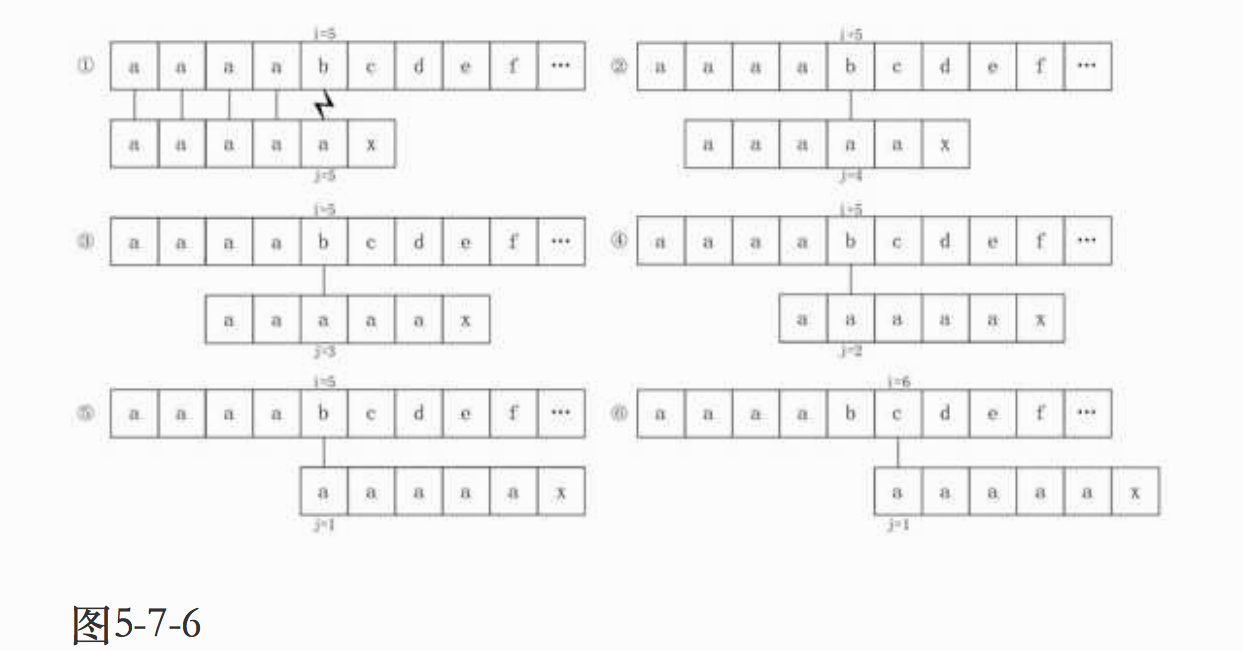# nextval数组值推导

1．T="ababaaaba"

j             123456789

next[j]          011234223
nextval[j]     010104210

1）当j=1时，nextval=0；

2）当j=2时，因第二位字符“b”的next值是1，而第一位就是“a”，它们不相等，所以nextval=next=1，维持原值。

3）当j=3时，因为第三位字符“a”的next值为1，所以与第一位的“a”比较得知它们相等，所以nextval=nextval=0；如图5-7-7所示。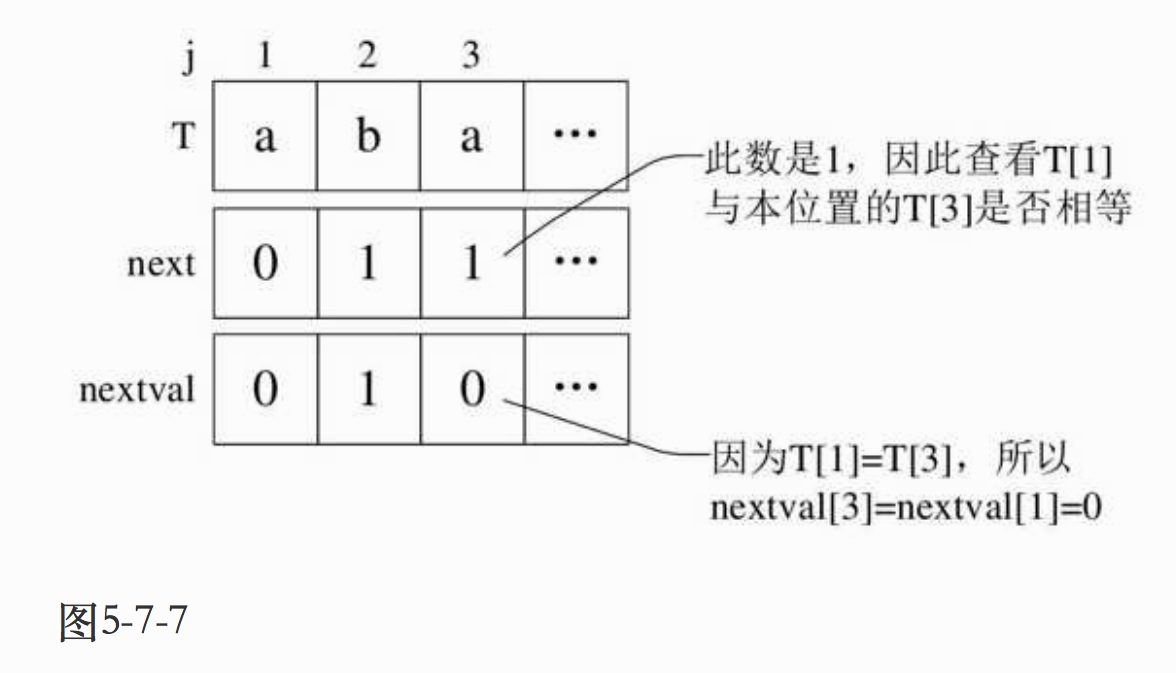4）当j=4时，第四位的字符“b”next值为2，所以与第二位的“b”相比较得到结果是相等，因此nextval=nextval=1；如图5-7-8所示。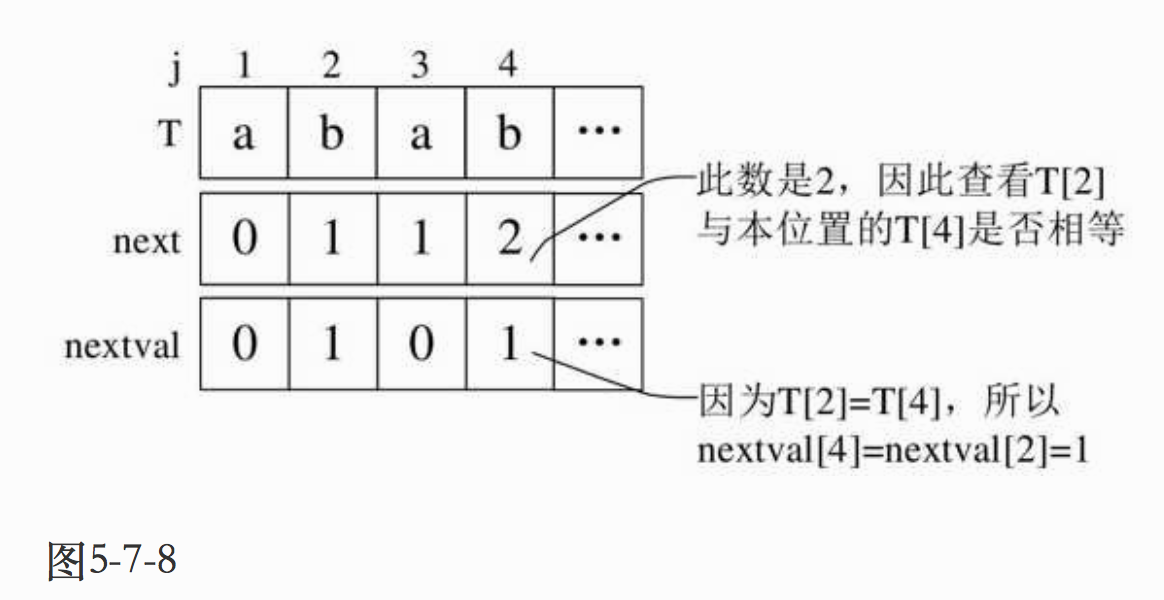5）当j=5时，next值为3，第五个字符“a”与第三个字符“a”相等，因此nextval=nextval=0；

6）当j=6时，next值为4，第六个字符“a”与第四个字符“b”不相等，因此nextval=4；

7）当j=7时，next值为2，第七个字符“a”与第二个字符“b”不相等，因此nextval=2；

8）当j=8时，next值为2，第八个字符“b”与第二个字符“b”相等，因此nextval=nextval=1；

9）当j=9时，next值为3，第九个字符“a”与第三个字符“a”相等，因此nextval=nextval=0。

2．T="aaaaaaaab"

j           123456789

next[j]        012345678
nextval[j]    000000008

1）当j=1时，nextval=0；

2）当j=2时，next值为1，第二个字符与第一个字符相等，所以nextval=nextval=0；

3）同样的道理，其后都为0……；

4）当j=9时，next值为8，第九个字符“b”与第八个字符“a”不相等，所以nextval=8。

kmp 大话数据结构 相关内容

2020-01-29 21:41:13 wangqing008 阅读数 30
• ###### 图解Java数据结构和算法

1.算法是程序的灵魂，优秀的程序在对海量数据处理时，依然保持高速计算，就需要高效的数据结构和算法支撑。2.网上数据结构和算法的课程不少，但存在两个问题： 1)授课方式单一，大多是照着代码念一遍，数据结构和算法本身就比较难理解，对基础好的学员来说，还好一点，对基础不好的学生来说，基本上就是听天书了 2)说是讲数据结构和算法，但大多是挂羊头卖狗肉，算法讲的很少。 本课程针对上述问题，有针对性的进行了升级  3)授课方式采用图解+算法游戏的方式，让课程生动有趣好理解 4)系统全面的讲解了数据结构和算法, 除常用数据结构和算法外，还包括程序员常用10大算法：二分查找算法（非递归）、分治算法、动态规划算法、KMP算法、贪心算法、普里姆算法、克鲁斯卡尔算法、迪杰斯特拉算法、弗洛伊德算法、马踏棋盘算法。可以解决面试遇到的最短路径、最小生成树、最小连通图、动态规划等问题及衍生出的面试题，让你秒杀其他面试小伙伴 3.如果你不想永远都是代码工人，就需要花时间来研究下数据结构和算法。教程内容： 本教程是使用Java来讲解数据结构和算法，考虑到数据结构和算法较难，授课采用图解加算法游戏的方式。内容包括: 稀疏数组、单向队列、环形队列、单向链表、双向链表、环形链表、约瑟夫问题、栈、前缀、中缀、后缀表达式、中缀表达式转换为后缀表达式、递归与回溯、迷宫问题、八皇后问题、算法的时间复杂度、冒泡排序、选择排序、插入排序、快速排序、归并排序、希尔排序、基数排序(桶排序)、堆排序、排序速度分析、二分查找、插值查找、斐波那契查找、散列、哈希表、二叉树、二叉树与数组转换、二叉排序树(BST)、AVL树、线索二叉树、赫夫曼树、赫夫曼编码、多路查找树(B树B+树和B*树)、图、图的DFS算法和BFS、程序员常用10大算法、二分查找算法(非递归)、分治算法、动态规划算法、KMP算法、贪心算法、普里姆算法、克鲁斯卡尔算法、迪杰斯特拉算法、弗洛伊德算法马踏棋盘算法。 学习目标：通过学习，学员能掌握主流数据结构和算法的实现机制，开阔编程思路，提高优化程序的能力。

5213 人正在学习 去看看 佟刚

### 1，正本书的结构### 2，几个内容整理

KMP算法

KMP算法前段时间也是终于能按自己的理解消化了一遍，比大一大二刚学的时候又理解了不少，其实断断续续也是看了好多遍。一直觉得KMP算法是比较精妙的，现在来看确实很经典。这本书并没有介绍的特别好，没有讲解的很透彻。

KMP算法使用在字符串查找里的一种算法，暴力的模板匹配是用子串去匹配待查找的串，一个字符一个字符的是比对，如果失败，则退回去重新从下一个字符开始匹配，但是很多时候我们不需要退回去，而是利用子串自身的信息，跳到下一个匹配点进行匹配。KMP的核心思想是如何利用好子串的已知信息进行匹配，如果能明白这一点，基本上KMP就理解了一半了。

1，计算子串的已知信息

2，利用子串已知信息去模板匹配，搜索

aaaaaaaab----->   -1 0 1 2 3 4 5 6 7

ababababab----->    -1 0 0 1 2 3 4 5 6 7

``````def kmp_find(src, dst):
next = kmp_next(dst)
i, j = 0, 0
while i < len(src) and j < len(dst):
if j == -1 or src[i] == dst[j]:
i = i + 1
j = j + 1
else:
j = next[j]
if j == len(dst):
return i - j
else:
return -1``````

``````def kmp_next(dst):
next =  * len(dst)
next = -1
i, j = 0, -1
while i < len(dst) - 1:
if j == -1 or dst[i] == dst[j]:
i = i + 1
j = j + 1
next[i] = j
else:
j = next[j]
return next``````

``````def kmp_next(dst):
next =  * len(dst)
next = -1
i, j = 0, -1
while i < len(dst) - 1:
if j == -1 or dst[i] == dst[j]:
i = i + 1
j = j + 1
# 只改动了这里
if dst[i] == dst[j]:
next[i] = next[j]
else:
next[i] = j
else:
j = next[j]
return next``````

kmp 大话数据结构 相关内容

2017-08-07 09:36:40 wgf5845201314 阅读数 160
• ###### 图解Java数据结构和算法

1.算法是程序的灵魂，优秀的程序在对海量数据处理时，依然保持高速计算，就需要高效的数据结构和算法支撑。2.网上数据结构和算法的课程不少，但存在两个问题： 1)授课方式单一，大多是照着代码念一遍，数据结构和算法本身就比较难理解，对基础好的学员来说，还好一点，对基础不好的学生来说，基本上就是听天书了 2)说是讲数据结构和算法，但大多是挂羊头卖狗肉，算法讲的很少。 本课程针对上述问题，有针对性的进行了升级  3)授课方式采用图解+算法游戏的方式，让课程生动有趣好理解 4)系统全面的讲解了数据结构和算法, 除常用数据结构和算法外，还包括程序员常用10大算法：二分查找算法（非递归）、分治算法、动态规划算法、KMP算法、贪心算法、普里姆算法、克鲁斯卡尔算法、迪杰斯特拉算法、弗洛伊德算法、马踏棋盘算法。可以解决面试遇到的最短路径、最小生成树、最小连通图、动态规划等问题及衍生出的面试题，让你秒杀其他面试小伙伴 3.如果你不想永远都是代码工人，就需要花时间来研究下数据结构和算法。教程内容： 本教程是使用Java来讲解数据结构和算法，考虑到数据结构和算法较难，授课采用图解加算法游戏的方式。内容包括: 稀疏数组、单向队列、环形队列、单向链表、双向链表、环形链表、约瑟夫问题、栈、前缀、中缀、后缀表达式、中缀表达式转换为后缀表达式、递归与回溯、迷宫问题、八皇后问题、算法的时间复杂度、冒泡排序、选择排序、插入排序、快速排序、归并排序、希尔排序、基数排序(桶排序)、堆排序、排序速度分析、二分查找、插值查找、斐波那契查找、散列、哈希表、二叉树、二叉树与数组转换、二叉排序树(BST)、AVL树、线索二叉树、赫夫曼树、赫夫曼编码、多路查找树(B树B+树和B*树)、图、图的DFS算法和BFS、程序员常用10大算法、二分查找算法(非递归)、分治算法、动态规划算法、KMP算法、贪心算法、普里姆算法、克鲁斯卡尔算法、迪杰斯特拉算法、弗洛伊德算法马踏棋盘算法。 学习目标：通过学习，学员能掌握主流数据结构和算法的实现机制，开阔编程思路，提高优化程序的能力。

5213 人正在学习 去看看 佟刚
```#include "string.h"
#include "stdio.h"
#include "stdlib.h"
#include "io.h"
#include "math.h"
#include "time.h"

#define OK 1
#define ERROR 0
#define TRUE 1
#define FALSE 0
#define MAXSIZE 100 /* 存储空间初始分配量 */

typedef int Status;		/* Status是函数的类型,其值是函数结果状态代码，如OK等 */
typedef int ElemType;	/* ElemType类型根据实际情况而定，这里假设为int */

typedef char String[MAXSIZE+1]; /*  0号单元存放串的长度 */

/* 生成一个其值等于chars的串T */
Status StrAssign(String T,char *chars)
{
int i;
if(strlen(chars)>MAXSIZE)
return ERROR;
else
{
T=strlen(chars);
for(i=1;i<=T;i++)
T[i]=*(chars+i-1);
return OK;
}
}

Status ClearString(String S)
{
S=0;/*  令串长为零 */
return OK;
}

/*  输出字符串T。 */
void StrPrint(String T)
{
int i;
for(i=1;i<=T;i++)
printf("%c",T[i]);
printf("\n");
}

/*  输出Next数组值。 */
void NextPrint(int next[],int length)
{
int i;
for(i=1;i<=length;i++)
printf("%d",next[i]);
printf("\n");
}

/* 返回串的元素个数 */
int StrLength(String S)
{
return S;
}

/* 朴素的模式匹配法 */
int Index(String S, String T, int pos)
{
int i = pos;	/* i用于主串S中当前位置下标值，若pos不为1，则从pos位置开始匹配 */
int j = 1;				/* j用于子串T中当前位置下标值 */
while (i <= S && j <= T) /* 若i小于S的长度并且j小于T的长度时，循环继续 */
{
if (S[i] == T[j]) 	/* 两字母相等则继续 */
{
++i;
++j;
}
else 				/* 指针后退重新开始匹配 */
{
i = i-j+2;		/* i退回到上次匹配首位的下一位 */
j = 1; 			/* j退回到子串T的首位 */
}
}
if (j > T)
return i-T;
else
return 0;
}

/* 通过计算返回子串T的next数组。 */
void get_next(String T, int *next)
{
int i,j;
i=1;
j=0;
next=0;
while (i<T)  /* 此处T表示串T的长度 */
{
if(j==0 || T[i]== T[j]) 	/* T[i]表示后缀的单个字符，T[j]表示前缀的单个字符 */
{
++i;
++j;
next[i] = j;
}
else
j= next[j];	/* 若字符不相同，则j值回溯 */
}
}

/* 返回子串T在主串S中第pos个字符之后的位置。若不存在，则函数返回值为0。 */
/*  T非空，1≤pos≤StrLength(S)。 */
int Index_KMP(String S, String T, int pos)
{
int i = pos;		/* i用于主串S中当前位置下标值，若pos不为1，则从pos位置开始匹配 */
int j = 1;			/* j用于子串T中当前位置下标值 */
int next;		/* 定义一next数组 */
get_next(T, next);	/* 对串T作分析，得到next数组 */
while (i <= S && j <= T) /* 若i小于S的长度并且j小于T的长度时，循环继续 */
{
if (j==0 || S[i] == T[j]) 	/* 两字母相等则继续，与朴素算法增加了j=0判断 */
{
++i;
++j;
}
else 			/* 指针后退重新开始匹配 */
j = next[j];/* j退回合适的位置，i值不变 */
}
if (j > T)
return i-T;
else
return 0;
}

/* 求模式串T的next函数修正值并存入数组nextval */
void get_nextval(String T, int *nextval)
{
int i,j;
i=1;
j=0;
nextval=0;
while (i<T)  /* 此处T表示串T的长度 */
{
if(j==0 || T[i]== T[j]) 	/* T[i]表示后缀的单个字符，T[j]表示前缀的单个字符 */
{
++i;
++j;
if (T[i]!=T[j])      /* 若当前字符与前缀字符不同 */
nextval[i] = j;	/* 则当前的j为nextval在i位置的值 */
else
nextval[i] = nextval[j];	/* 如果与前缀字符相同，则将前缀字符的 */
/* nextval值赋值给nextval在i位置的值 */
}
else
j= nextval[j];			/* 若字符不相同，则j值回溯 */
}
}

int Index_KMP1(String S, String T, int pos)
{
int i = pos;		/* i用于主串S中当前位置下标值，若pos不为1，则从pos位置开始匹配 */
int j = 1;			/* j用于子串T中当前位置下标值 */
int next;		/* 定义一next数组 */
get_nextval(T, next);	/* 对串T作分析，得到next数组 */
while (i <= S && j <= T) /* 若i小于S的长度并且j小于T的长度时，循环继续 */
{
if (j==0 || S[i] == T[j]) 	/* 两字母相等则继续，与朴素算法增加了j=0判断 */
{
++i;
++j;
}
else 			/* 指针后退重新开始匹配 */
j = next[j];/* j退回合适的位置，i值不变 */
}
if (j > T)
return i-T;
else
return 0;
}

int main()
{
int i,*p;
String s1,s2;

StrAssign(s1,"abcdex");
printf("子串为: ");
StrPrint(s1);
i=StrLength(s1);
p=(int*)malloc((i+1)*sizeof(int));
get_next(s1,p);
printf("Next为: ");
NextPrint(p,StrLength(s1));
printf("\n");

StrAssign(s1,"abcabx");
printf("子串为: ");
StrPrint(s1);
i=StrLength(s1);
p=(int*)malloc((i+1)*sizeof(int));
get_next(s1,p);
printf("Next为: ");
NextPrint(p,StrLength(s1));
printf("\n");

StrAssign(s1,"ababaaaba");
printf("子串为: ");
StrPrint(s1);
i=StrLength(s1);
p=(int*)malloc((i+1)*sizeof(int));
get_next(s1,p);
printf("Next为: ");
NextPrint(p,StrLength(s1));
printf("\n");

StrAssign(s1,"aaaaaaaab");
printf("子串为: ");
StrPrint(s1);
i=StrLength(s1);
p=(int*)malloc((i+1)*sizeof(int));
get_next(s1,p);
printf("Next为: ");
NextPrint(p,StrLength(s1));
printf("\n");

StrAssign(s1,"ababaaaba");
printf("   子串为: ");
StrPrint(s1);
i=StrLength(s1);
p=(int*)malloc((i+1)*sizeof(int));
get_next(s1,p);
printf("   Next为: ");
NextPrint(p,StrLength(s1));
get_nextval(s1,p);
printf("NextVal为: ");
NextPrint(p,StrLength(s1));
printf("\n");

StrAssign(s1,"aaaaaaaab");
printf("   子串为: ");
StrPrint(s1);
i=StrLength(s1);
p=(int*)malloc((i+1)*sizeof(int));
get_next(s1,p);
printf("   Next为: ");
NextPrint(p,StrLength(s1));
get_nextval(s1,p);
printf("NextVal为: ");
NextPrint(p,StrLength(s1));

printf("\n");

StrAssign(s1,"00000000000000000000000000000000000000000000000001");
printf("主串为: ");
StrPrint(s1);
StrAssign(s2,"0000000001");
printf("子串为: ");
StrPrint(s2);
printf("\n");
printf("主串和子串在第%d个字符处首次匹配（朴素模式匹配算法）\n",Index(s1,s2,1));
printf("主串和子串在第%d个字符处首次匹配（KMP算法） \n",Index_KMP(s1,s2,1));
printf("主串和子串在第%d个字符处首次匹配（KMP改良算法） \n",Index_KMP1(s1,s2,1));

return 0;
}
```kmp 大话数据结构 相关内容